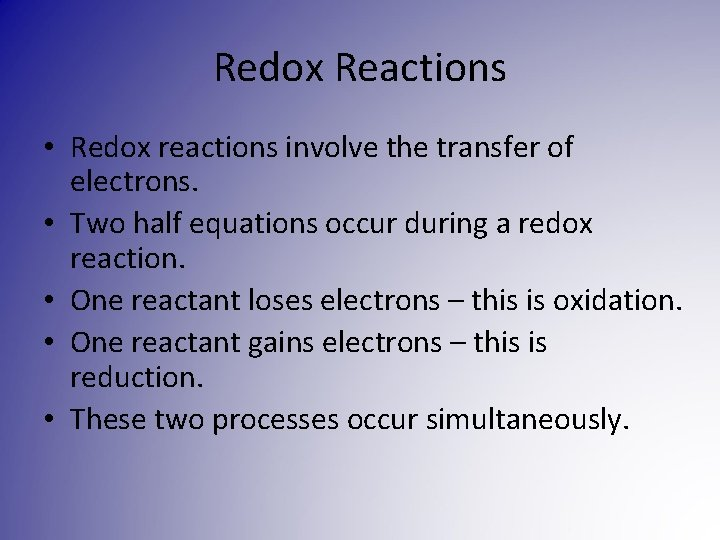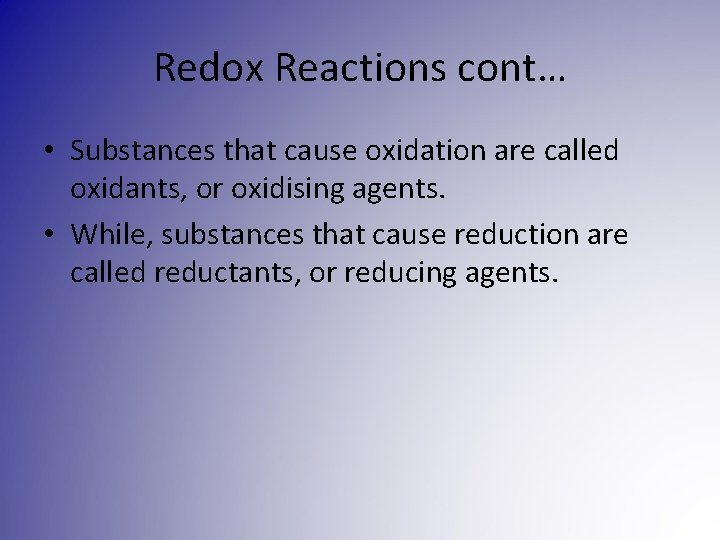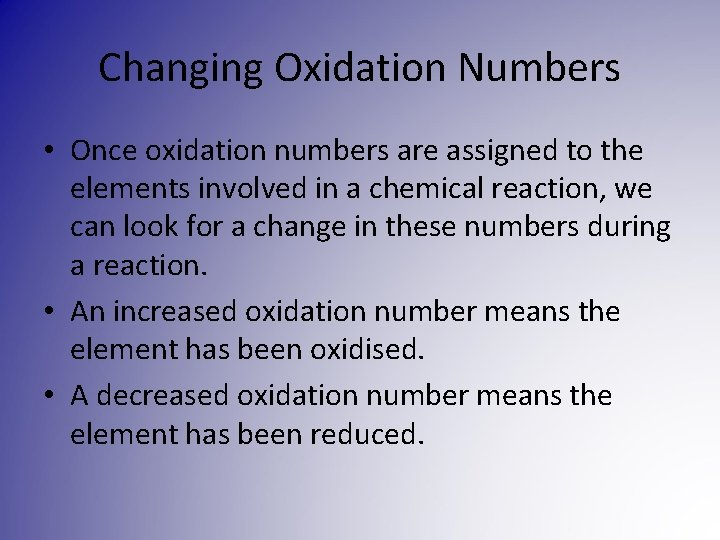# Week 3 Lesson 1 Chapter 5 Analysing Oxidants

• Slides: 9Week 3, Lesson 1 Chapter 5 – Analysing Oxidants & ReductantsRedox Reactions • Redox reactions involve the transfer of electrons. • Two half equations occur during a redox reaction. • One reactant loses electrons – this is oxidation. • One reactant gains electrons – this is reduction. • These two processes occur simultaneously.Redox Reactions cont… • Substances that cause oxidation are called oxidants, or oxidising agents. • While, substances that cause reduction are called reductants, or reducing agents.Oxidation Numbers • To determine whether a reaction is a redox reaction, oxidation numbers are assigned to elements involved in the reaction. • These numbers are determined by applying a set of rules that treat molecular substances as if they were composed of ions. • Oxidation numbers have no physical meaning. • Oxidation numbers are also known as oxidation states.Oxidation Number Rules • Free elements have an oxidation number of 0. • In ionic compounds composed of simple ions, the oxidation number is the same as the charge on that ion. • Some elements have oxidation numbers that are regarded as fixed, except in a few exceptional circumstances: – Oxygen usually takes -2 in compounds, except in peroxides it is -1. – Hydrogen takes +1 in compounds, except in metal hydrides if is -1. • The sum of the oxidation number in a neutral compound is zero. • In a polyatomic ion the sum of the oxidation numbers is equal to the charge on that ion. • The most electronegative element in a compound has the negative oxidation number.Variable Oxidation Number • In all their compounds, the group I metals only have an oxidation number of +1 and the group II metals only have an oxidation number of +2. • The oxidation numbers of transition metals vary depending on the compound. • The oxidation numbers of non-metallic elements may also vary from compound to compound.Changing Oxidation Numbers • Once oxidation numbers are assigned to the elements involved in a chemical reaction, we can look for a change in these numbers during a reaction. • An increased oxidation number means the element has been oxidised. • A decreased oxidation number means the element has been reduced.Writing Half Equations • Half equations that involve atoms or simple ions can be written with little difficulty, as you just need to add electrons to the appropriate side. • Half equations involving polyatomic ions is a little more difficult. • The process is as follows: 1. Balance all elements except hydrogen and oxygen in the half equation. 2. Balance the oxygen atoms by adding water. 3. Balance the hydrogen atoms by adding H+ ions. 4. Balance the charge by adding electrons.Volumetric Analysis • This process can also be used to find the concentration of solutions of substances in a redox reaction. • Instead of reacting an acid with a base, a redox titration involves reaction of an oxidant with a reductant. • To find the concentration, the same process is followed as in an acid-base reaction.